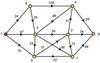shortest path

SPFA算法、Dijkstra算法等

• ## 最短路径问题---Dijkstra算法详解

万次阅读 多人点赞 2017-03-08 16:42:46
前言 Nobody can go back and start a new beginning,but anyone can start today and make a new ...从图中的某个顶点出发到达另外一个顶点的所经过的边的权重和最小的一条路径，称为最短路径 解决问题的算法：...
前言
Nobody can go back and start a new beginning,but anyone can start today and make a new ending.
Name:Willam
Time:2017/3/8

1、最短路径问题介绍

问题解释：
从图中的某个顶点出发到达另外一个顶点的所经过的边的权重和最小的一条路径，称为最短路径

解决问题的算法：

迪杰斯特拉算法（Dijkstra算法）
弗洛伊德算法（Floyd算法）
SPFA算法

这篇博客，我们就对Dijkstra算法来做一个详细的介绍

2、Dijkstra算法介绍

算法特点：

迪科斯彻算法使用了广度优先搜索解决赋权有向图或者无向图的单源最短路径问题，算法最终得到一个最短路径树。该算法常用于路由算法或者作为其他图算法的一个子模块。
算法的思路

Dijkstra算法采用的是一种贪心的策略，声明一个数组dis来保存源点到各个顶点的最短距离和一个保存已经找到了最短路径的顶点的集合：T，初始时，原点 s 的路径权重被赋为 0 （dis[s] = 0）。若对于顶点 s 存在能直接到达的边（s,m），则把dis[m]设为w（s, m）,同时把所有其他（s不能直接到达的）顶点的路径长度设为无穷大。初始时，集合T只有顶点s。
然后，从dis数组选择最小值，则该值就是源点s到该值对应的顶点的最短路径，并且把该点加入到T中，OK，此时完成一个顶点，
然后，我们需要看看新加入的顶点是否可以到达其他顶点并且看看通过该顶点到达其他点的路径长度是否比源点直接到达短，如果是，那么就替换这些顶点在dis中的值。
然后，又从dis中找出最小值，重复上述动作，直到T中包含了图的所有顶点。

3、Dijkstra算法示例演示

下面我求下图，从顶点v1到其他各个顶点的最短路径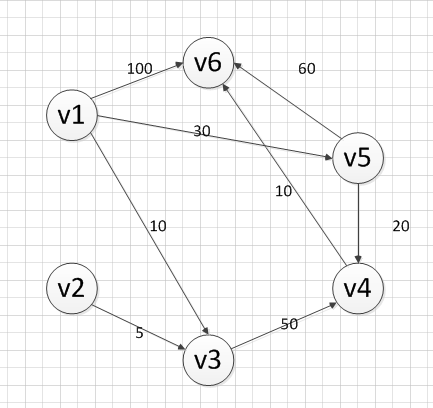首先第一步，我们先声明一个dis数组，该数组初始化的值为：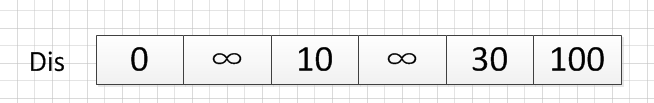我们的顶点集T的初始化为：T={v1}

既然是求 v1顶点到其余各个顶点的最短路程，那就先找一个离 1 号顶点最近的顶点。通过数组 dis 可知当前离v1顶点最近是 v3顶点。当选择了 2 号顶点后，dis（下标从0开始）的值就已经从“估计值”变为了“确定值”，即 v1顶点到 v3顶点的最短路程就是当前 dis值。将V3加入到T中。
为什么呢？因为目前离 v1顶点最近的是 v3顶点，并且这个图所有的边都是正数，那么肯定不可能通过第三个顶点中转，使得 v1顶点到 v3顶点的路程进一步缩短了。因为 v1顶点到其它顶点的路程肯定没有 v1到 v3顶点短.

OK，既然确定了一个顶点的最短路径，下面我们就要根据这个新入的顶点V3会有出度，发现以v3 为弧尾的有： < v3,v4 >,那么我们看看路径：v1–v3–v4的长度是否比v1–v4短，其实这个已经是很明显的了，因为dis代表的就是v1–v4的长度为无穷大，而v1–v3–v4的长度为：10+50=60，所以更新dis的值,得到如下结果：因此 dis要更新为 60。这个过程有个专业术语叫做“松弛”。即 v1顶点到 v4顶点的路程即 dis，通过 < v3,v4> 这条边松弛成功。这便是 Dijkstra 算法的主要思想：通过“边”来松弛v1顶点到其余各个顶点的路程。

然后，我们又从除dis和dis外的其他值中寻找最小值，发现dis的值最小，通过之前是解释的原理，可以知道v1到v5的最短距离就是dis的值，然后，我们把v5加入到集合T中，然后，考虑v5的出度是否会影响我们的数组dis的值，v5有两条出度：< v5,v4>和 < v5,v6>,然后我们发现：v1–v5–v4的长度为：50，而dis的值为60，所以我们要更新dis的值.另外，v1-v5-v6的长度为：90，而dis为100，所以我们需要更新dis的值。更新后的dis数组如下图：然后，继续从dis中选择未确定的顶点的值中选择一个最小的值，发现dis的值是最小的，所以把v4加入到集合T中，此时集合T={v1,v3,v5,v4},然后，考虑v4的出度是否会影响我们的数组dis的值，v4有一条出度：< v4,v6>,然后我们发现：v1–v5–v4–v6的长度为：60，而dis的值为90，所以我们要更新dis的值，更新后的dis数组如下图：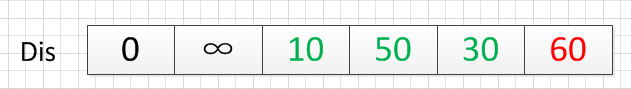然后，我们使用同样原理，分别确定了v6和v2的最短路径，最后dis的数组的值如下：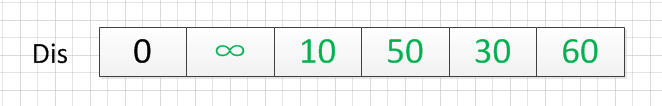因此，从图中，我们可以发现v1-v2的值为：∞，代表没有路径从v1到达v2。所以我们得到的最后的结果为：

起点  终点    最短路径    长度
v1    v2     无          ∞
v3     {v1,v3}    10
v4     {v1,v5,v4}  50
v5     {v1,v5}    30
v6     {v1，v5,v4,v6} 60


4、Dijkstra算法的代码实现（c++）

Dijkstra.h文件的代码

/************************************************************/
/*                程序作者：Willam                          */
/*                程序完成时间：2017/3/8                    */
/*                有任何问题请联系：2930526477@qq.com       */
/************************************************************/
//@尽量写出完美的程序

#pragma once
//#pragma once是一个比较常用的C/C++杂注，
//只要在头文件的最开始加入这条杂注，
//就能够保证头文件只被编译一次。

#include<iostream>
#include<string>
using namespace std;

/*
本程序是使用Dijkstra算法实现求解最短路径的问题
采用的邻接矩阵来存储图
*/
//记录起点到每个顶点的最短路径的信息
struct Dis {
string path;
int value;
bool visit;
Dis() {
visit = false;
value = 0;
path = "";
}
};

class Graph_DG {
private:
int vexnum;   //图的顶点个数
int edge;     //图的边数
int **arc;   //邻接矩阵
Dis * dis;   //记录各个顶点最短路径的信息
public:
//构造函数
Graph_DG(int vexnum, int edge);
//析构函数
~Graph_DG();
// 判断我们每次输入的的边的信息是否合法
//顶点从1开始编号
bool check_edge_value(int start, int end, int weight);
//创建图
void createGraph();
//打印邻接矩阵
void print();
//求最短路径
void Dijkstra(int begin);
//打印最短路径
void print_path(int);
};


Dijkstra.cpp文件的代码

#include"Dijkstra.h"

//构造函数
Graph_DG::Graph_DG(int vexnum, int edge) {
//初始化顶点数和边数
this->vexnum = vexnum;
this->edge = edge;
//为邻接矩阵开辟空间和赋初值
arc = new int*[this->vexnum];
dis = new Dis[this->vexnum];
for (int i = 0; i < this->vexnum; i++) {
arc[i] = new int[this->vexnum];
for (int k = 0; k < this->vexnum; k++) {
//邻接矩阵初始化为无穷大
arc[i][k] = INT_MAX;
}
}
}
//析构函数
Graph_DG::~Graph_DG() {
delete[] dis;
for (int i = 0; i < this->vexnum; i++) {
delete this->arc[i];
}
delete arc;
}

// 判断我们每次输入的的边的信息是否合法
//顶点从1开始编号
bool Graph_DG::check_edge_value(int start, int end, int weight) {
if (start<1 || end<1 || start>vexnum || end>vexnum || weight < 0) {
return false;
}
return true;
}

void Graph_DG::createGraph() {
cout << "请输入每条边的起点和终点（顶点编号从1开始）以及其权重" << endl;
int start;
int end;
int weight;
int count = 0;
while (count != this->edge) {
cin >> start >> end >> weight;
//首先判断边的信息是否合法
while (!this->check_edge_value(start, end, weight)) {
cout << "输入的边的信息不合法，请重新输入" << endl;
cin >> start >> end >> weight;
}
//对邻接矩阵对应上的点赋值
arc[start - 1][end - 1] = weight;
//无向图添加上这行代码
//arc[end - 1][start - 1] = weight;
++count;
}
}

void Graph_DG::print() {
cout << "图的邻接矩阵为：" << endl;
int count_row = 0; //打印行的标签
int count_col = 0; //打印列的标签
//开始打印
while (count_row != this->vexnum) {
count_col = 0;
while (count_col != this->vexnum) {
if (arc[count_row][count_col] == INT_MAX)
cout << "∞" << " ";
else
cout << arc[count_row][count_col] << " ";
++count_col;
}
cout << endl;
++count_row;
}
}
void Graph_DG::Dijkstra(int begin){
//首先初始化我们的dis数组
int i;
for (i = 0; i < this->vexnum; i++) {
//设置当前的路径
dis[i].path = "v" + to_string(begin) + "-->v" + to_string(i + 1);
dis[i].value = arc[begin - 1][i];
}
//设置起点的到起点的路径为0
dis[begin - 1].value = 0;
dis[begin - 1].visit = true;

int count = 1;
//计算剩余的顶点的最短路径（剩余this->vexnum-1个顶点）
while (count != this->vexnum) {
//temp用于保存当前dis数组中最小的那个下标
//min记录的当前的最小值
int temp=0;
int min = INT_MAX;
for (i = 0; i < this->vexnum; i++) {
if (!dis[i].visit && dis[i].value<min) {
min = dis[i].value;
temp = i;
}
}
//cout << temp + 1 << "  "<<min << endl;
//把temp对应的顶点加入到已经找到的最短路径的集合中
dis[temp].visit = true;
++count;
for (i = 0; i < this->vexnum; i++) {
//注意这里的条件arc[temp][i]!=INT_MAX必须加，不然会出现溢出，从而造成程序异常
if (!dis[i].visit && arc[temp][i]!=INT_MAX && (dis[temp].value + arc[temp][i]) < dis[i].value) {
//如果新得到的边可以影响其他为访问的顶点，那就就更新它的最短路径和长度
dis[i].value = dis[temp].value + arc[temp][i];
dis[i].path = dis[temp].path + "-->v" + to_string(i + 1);
}
}
}

}
void Graph_DG::print_path(int begin) {
string str;
str = "v" + to_string(begin);
cout << "以"<<str<<"为起点的图的最短路径为：" << endl;
for (int i = 0; i != this->vexnum; i++) {
if(dis[i].value!=INT_MAX)
cout << dis[i].path << "=" << dis[i].value << endl;
else {
cout << dis[i].path << "是无最短路径的" << endl;
}
}
}

main.cpp文件的代码

#include"Dijkstra.h"

//检验输入边数和顶点数的值是否有效，可以自己推算为啥：
//顶点数和边数的关系是：((Vexnum*(Vexnum - 1)) / 2) < edge
bool check(int Vexnum, int edge) {
if (Vexnum <= 0 || edge <= 0 || ((Vexnum*(Vexnum - 1)) / 2) < edge)
return false;
return true;
}
int main() {
int vexnum; int edge;

cout << "输入图的顶点个数和边的条数：" << endl;
cin >> vexnum >> edge;
while (!check(vexnum, edge)) {
cout << "输入的数值不合法，请重新输入" << endl;
cin >> vexnum >> edge;
}
Graph_DG graph(vexnum, edge);
graph.createGraph();
graph.print();
graph.Dijkstra(1);
graph.print_path(1);
system("pause");
return 0;
}

输入：

6 8
1 3 10
1 5 30
1 6 100
2 3 5
3 4 50
4 6 10
5 6 60
5 4 20

输出：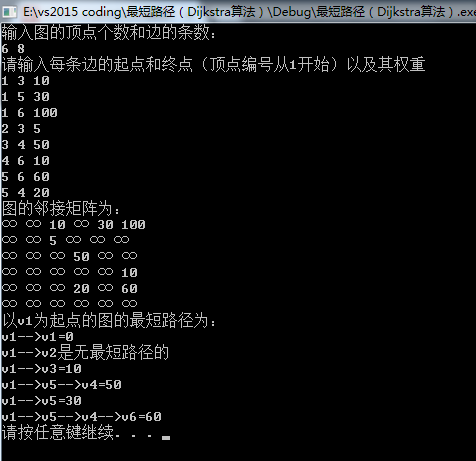从输出可以看出，程序的结果和我们之前手动计算的结果是一样的。
展开全文算法 数据结构 Dijkstra算法
• 最短路径： 在一个带权图中，顶点V0到图中任意一个顶点Vi的一条路径所经过边上的权值之和，定义为该路径的带权路径长度，把带权路径最短的那条路径称为最短路径。 DiskStra算法： 求单源最短路径，即求一个顶点到...
最短路径：

在一个带权图中，顶点V0到图中任意一个顶点Vi的一条路径所经过边上的权值之和，定义为该路径的带权路径长度，把带权路径最短的那条路径称为最短路径。

DiskStra算法：

求单源最短路径，即求一个顶点到任意顶点的最短路径，其时间复杂度为O(V*V)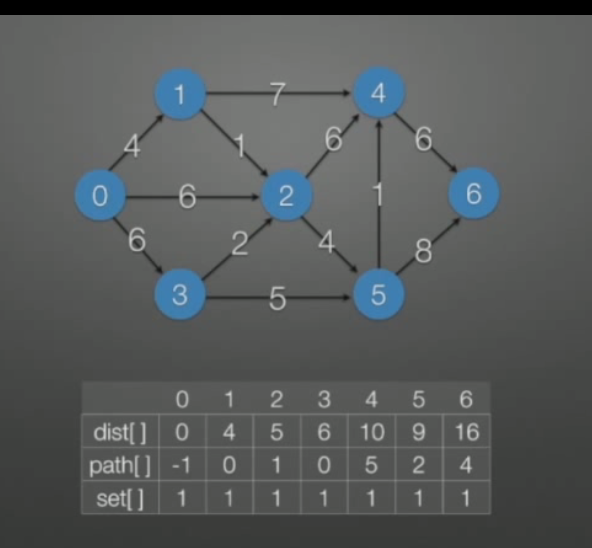如图所示：求顶点0到各顶点之间的最短路径

代码实现：

#include<stdio.h>
#include<stdlib.h>
#define MaxVexNum 50
#define MaxInt 32767
#define MaxEdgeNum 50
//邻接矩阵
typedef int VertexType;
typedef int EdgeType;
typedef struct AMGraph{
VertexType vexs[MaxVexNum];//顶点表
EdgeType arcs[MaxVexNum][MaxVexNum];//邻接矩阵表
int vexnum,edgenum;//顶点数，边数
}AMGraph;

void createGraph(AMGraph &g){//创建无向图
printf("请输入顶点数：");
scanf("%d",&g.vexnum);
printf("\n请输入边数：");
scanf("%d",&g.edgenum);

//初始化顶点表
for(int i=0;i<g.vexnum;i++){
g.vexs[i]=i;
}
for(int i=0;i<g.vexnum;i++){
for(int j=0;j<g.vexnum;j++){
g.arcs[i][j]=MaxInt;
if(i==j) g.arcs[i][j]=0;
}
}
printf("请输入边的信息以及边的权值(顶点是0~n-1)\n");
for(int i=0;i<g.edgenum;i++){
int x,y,w;
scanf("%d%d%d",&x,&y,&w);
g.arcs[x][y]=w;
//g.arcs[y][x]=w;
}
}
void PrintGraph(AMGraph g){
printf("邻接矩阵为:\n");
for(int i=0;i<g.vexnum;i++) {
printf("  %d",g.vexs[i]);
}
printf("\n");
for(int i=0;i<g.vexnum;i++){
printf("%d ",g.vexs[i]);
for(int j=0;j<g.vexnum;j++){
if(g.arcs[i][j]==32767){
printf("∞ ");
}else{
printf("%d  ",g.arcs[i][j]);
}
}
printf("\n");
}
}
//Dijkstra算法，求单源最短路径
void Dijkstra(AMGraph g,int dist[],int path[],int v0){
int n=g.vexnum,v;
int set[n];//set数组用于记录该顶点是否归并
//第一步：初始化
for(int i=0;i<n;i++){
set[i]=0;
dist[i]=g.arcs[v0][i];
if(dist[i]<MaxInt){//若距离小于MaxInt说明两点之间有路可通
path[i]=v0;//则更新路径i的前驱为v
}else{
path[i]=-1; //表示这两点之间没有边
}
}
set[v0]=1;//将初始顶点并入
path[v0]=-1;//初始顶点没有前驱

//第二步
for(int i=1;i<n;i++){//共n-1个顶点
int min=MaxInt;
//第二步：从i=1开始依次选一个距离顶点的最近顶点
for(int j=0;j<n;j++){
if(set[j]==0&&dist[j]<min){
v=j;
min=dist[j];
}
}
//将顶点并入
set[v]=1;
//第三步：在将新结点并入后，其初始顶点v0到各顶点的距离将会发生变化，所以需要更新dist[]数组
for(int j=0;j<n;j++){
if(set[j]==0&&dist[v]+g.arcs[v][j]<dist[j]){
dist[j]=dist[v]+g.arcs[v][j];
path[j]=v;
}
}
}
//输出
printf("       ");
for(int i=0;i<n;i++) printf("%d  ",i);

printf("\ndist[]:");
for(int i=0;i<n;i++) printf("%d  ",dist[i]);

printf("\npath[]:");
for(int i=0;i<n;i++) printf("%d  ",path[i]);

}

int main(){
AMGraph g;
createGraph(g);
int dist[g.vexnum];
int path[g.vexnum];
Dijkstra(g,dist,path,0);
}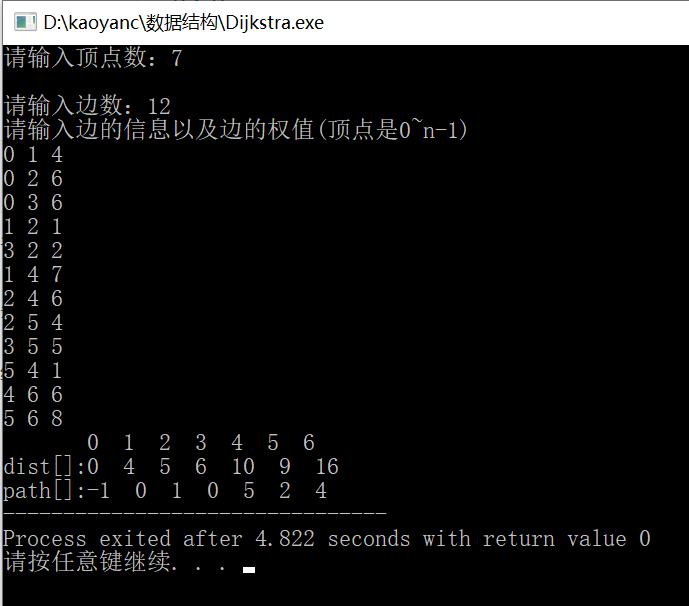Floyd算法：

求各顶点之间的最短路径,其时间复杂度为O(V*V*V)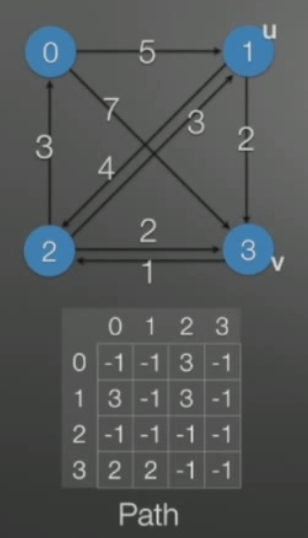如图所示，求<1,0>之间的最短路径：

代码实现：

#include<stdio.h>
#include<stdlib.h>
#define MaxVexNum 50
#define MaxInt 32767
#define MaxEdgeNum 50
//邻接矩阵
typedef int VertexType;
typedef int EdgeType;
typedef struct AMGraph{
VertexType vexs[MaxVexNum];//顶点表
EdgeType arcs[MaxVexNum][MaxVexNum];//邻接矩阵表
int vexnum,edgenum;//顶点数，边数
}AMGraph;

void createGraph(AMGraph &g){//创建无向图
printf("请输入顶点数：");
scanf("%d",&g.vexnum);
printf("\n请输入边数：");
scanf("%d",&g.edgenum);

//初始化顶点表
for(int i=0;i<g.vexnum;i++){
g.vexs[i]=i;
}
for(int i=0;i<g.vexnum;i++){
for(int j=0;j<g.vexnum;j++){
g.arcs[i][j]=MaxInt;
if(i==j) g.arcs[i][j]=0;
}
}
printf("请输入边的信息以及边的权值(顶点是0~n-1)\n");
for(int i=0;i<g.edgenum;i++){
int x,y,w;
scanf("%d%d%d",&x,&y,&w);
g.arcs[x][y]=w;
//g.arcs[y][x]=w;
}
}
void PrintGraph(AMGraph g){
printf("邻接矩阵为:\n");
for(int i=0;i<g.vexnum;i++) {
printf("  %d",g.vexs[i]);
}
printf("\n");
for(int i=0;i<g.vexnum;i++){
printf("%d ",g.vexs[i]);
for(int j=0;j<g.vexnum;j++){
if(g.arcs[i][j]==32767){
printf("∞ ");
}else{
printf("%d  ",g.arcs[i][j]);
}
}
printf("\n");
}
}

//Floyd算法

//递归输出两个顶点直接最短路径
void printPath(int u,int v,int path[][MaxVexNum]){
if(path[u][v]==-1){
printf("[%d %d] ",u,v);
}else{
int mid=path[u][v];
printPath(u,mid,path);
printPath(mid,v,path);
}
}
void Floyd(AMGraph g,int path[][MaxVexNum]){
int n=g.vexnum;
int A[n][n];
//第一步：初始化path[][]和A[][]数组
for(int i=0;i<n;i++){
for(int j=0;j<n;j++){
A[i][j]=g.arcs[i][j];
path[i][j]=-1;
}
}
//第二步：三重循环，寻找最短路径
for(int v=0;v<n;v++){//第一层是代表中间结点
for(int i=0;i<n;i++){
for(int j=0;j<n;j++){
if(A[i][j]>A[i][v]+A[v][j]){
A[i][j]=A[i][v]+A[v][j];
path[i][j]=v;
}
}
}
}
}

int main(){
AMGraph g;
createGraph(g);
PrintGraph(g);
int path[MaxVexNum][MaxVexNum];
Floyd(g,path);

printPath(1,0,path);
} 

代码运行截图：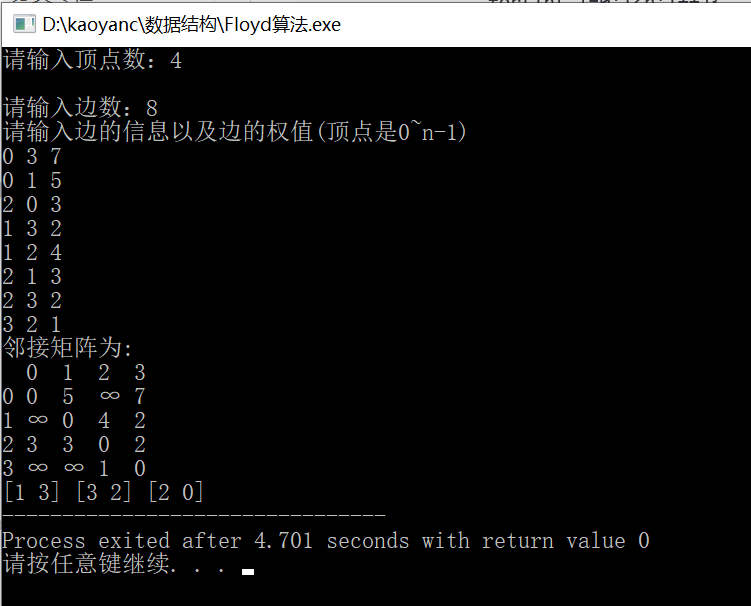展开全文• ## 图的五种最短路径算法

万次阅读 多人点赞 2018-03-17 17:02:49
本文总结了图的几种最短路径算法的实现：深度或广度优先搜索算法，费罗伊德算法，迪杰斯特拉算法，Bellman-Ford 算法。1）深度或广度优先搜索算法（解决单源最短路径）从起点开始访问所有深度遍历路径或广度优先路径...
本文总结了图的几种最短路径算法的实现：深度或广度优先搜索算法，费罗伊德算法，迪杰斯特拉算法，Bellman-Ford 算法。1）深度或广度优先搜索算法（解决单源最短路径）从起点开始访问所有深度遍历路径或广度优先路径，则到达终点节点的路径有多条，取其中路径权值最短的一条则为最短路径。下面是核心代码：void dfs(int cur,int dst){
if(minpath<dst) return;//当前走过的路径大雨之前的最短路径，没有必要再走下去了
if(cur==en){//临界条件，当走到终点n
if(minpath>dst){
minpath=dst;
return;
}
}
for(int i=1;i<=n;i++){
if(mark[i]==0&&edge[cur][i]!=inf&&edge[cur][i]!=0){
mark[i]=1;
dfs(i,dst+edge[cur][i]);
mark[i]=0;//需要在深度遍历返回时将访问标志置0
}
}
return;
}例：先输入n个节点，m条边，之后输入有向图的m条边，边的前两个元素表示起点和终点，第三个值表示权值，输出1号城市到n号城市的最短距离。#include<bits/stdc++.h>
using namespace std;
#define nmax 110
#define inf 999999999
int minpath,n,m,en,edge[nmax][nmax],mark[nmax];//最短路径，节点数，边数，终点，邻接矩阵，节点访问标记
void dfs(int cur,int dst){
if(minpath<dst) return;//当前走过的路径大雨之前的最短路径，没有必要再走下去了
if(cur==en){//临界条件，当走到终点n
if(minpath>dst){
minpath=dst;
return;
}
}
for(int i=1;i<=n;i++){
if(mark[i]==0&&edge[cur][i]!=inf&&edge[cur][i]!=0){
mark[i]=1;
dfs(i,dst+edge[cur][i]);
mark[i]=0;//需要在深度遍历返回时将访问标志置0
}
}
return;
}
int main ()
{
while(cin>>n>>m&&n!=0){
//初始化邻接矩阵
for(int i=1;i<=n;i++){
for(int j=1;j<=n;j++){
edge[i][j]=inf;
}
edge[i][i]=0;
}
int a,b;
while(m--){
cin>>a>>b;
cin>>edge[a][b];
}
minpath=inf;
memset(mark,0,sizeof(mark));
mark=1;
en=n;
dfs(1,0);
cout<<minpath<<endl;
}
}
程序运行结果如下：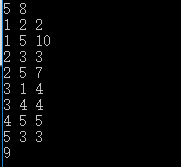2）弗洛伊德算法（解决多源最短路径）：时间复杂度o(n^3),空间复杂度o(n^2)基本思想：最开始只允许经过1号顶点进行中转，接下来只允许经过1号和2号顶点进行中转.......允许经过1~n号所有顶点进行中转，来不断动态更新任意两点之间的最短距离。即求从i号顶点到j顶点只经过前k号点的最短距离。分析如下：1，首先构建邻接矩阵edge[n+1][n+1],假如现在只允许经过1号节点，求任意两点间的最短距离，很显然edge[i][j]=min(edge[i][j],edge[i]+edge[j]),代码如下：for(int i=1;i<=n;i++){
for(int j=1;j<=n;j++){
if(edge[i][j]>edge[i]+edge[j]){
edge[i][j]=edge[i]+edge[j];
}
}
}2.接下来继续求在只允许经过1和2号两个顶点的情况下任意两点之间的最短距离，在已经实现了从i号顶点到j号顶点只经过前1号点的最短路程的前提下，现在插入第2号节点，来看看能不能更新最短路径，因此只需在步骤一求得的基础上，进行edge[i][j]=min(edge[i][j],edge[i]+edge[j]);.......3.很显然，需要n次这样的更新，表示依次插入了1号2号.......n号节点，最后求得的edge[i][j]是从i号顶点到j号顶点只经过前n号点的最短路程。因此核心代码如下：#include<bits/stdc++.h>
using namespace std;
#define inf 999999999
int main ()
{
for(int k=1;k<=n;k++){
for(int i=1;i<=n;i++){
for(int j=1;j<=n;j++){
if(edge[k][j]<inf&&edge[i][k]<inf&&edge[i][j]>edge[i][k]+edge[k][j]){
edge[i][j]=edge[i][k]+edge[k][j];
}
}
}
}
}
例1：寻找最短的从商场到赛场的路线。其中商店在1号节点处，赛场在n号节点处，1~n节点中有m条线路双向连接。/***先输入n，m，在输入m个三元组，n为路口数，m表示有几条路，其中1为商店，n为赛场，三元组分别表示起点终点，和该路径长，输出1到n的最短距离***/#include<bits/stdc++.h>
using namespace std;
#define inf 999999999
#define nmax 110
int n,m,edge[nmax][nmax];
int main ()
{
int a,b;
while(cin>>n>>m&&n!=0){
for(int i=1;i<=n;i++){
for(int j=1;j<=n;j++){
edge[i][j]=inf;
}
edge[i][i]=0;
}
while(m--){
cin>>a>>b;
cin>>edge[a][b];
edge[b][a]=edge[a][b];
}
for(int k=1;k<=n;k++){
for(int i=1;i<=n;i++){
for(int j=1;j<=n;j++){
if(edge[k][j]<inf&&edge[i][k]<inf&&edge[i][j]>edge[i][k]+edge[k][j]){
edge[i][j]=edge[i][k]+edge[k][j];
}
}
}
}
cout<<edge[n]<<endl;
}
}
程序运行结果如下：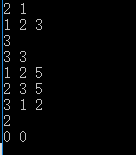3）迪杰斯特拉算法（解决单源最短路径）基本思想：每次找到离源点（如1号节点）最近的一个顶点，然后以该顶点为中心进行扩展，最终得到源点到其余所有点的最短路径。基本步骤：1，设置标记数组book[]：将所有的顶点分为两部分，已知最短路径的顶点集合P和未知最短路径的顶点集合Q，很显然最开始集合P只有源点一个顶点。book[i]为1表示在集合P中；2，设置最短路径数组dst[]并不断更新：初始状态下，dst[i]=edge[s][i](s为源点，edge为邻接矩阵),很显然此时dst[s]=0,book[s]=1.此时，在集合Q中可选择一个离源点s最近的顶点u加入到P中。并依据以u为新的中心点，对每一条边进行松弛操作（松弛是指由顶点s-->j的途中可以经过点u，并令dst[j]=min(dst[j],dst[u]+edge[u][j])）,并令book[u]=1;3，在集合Q中再次选择一个离源点s最近的顶点v加入到P中。并依据v为新的中心点，对每一条边进行松弛操作（即dst[j]=min(dst[j],dst[v]+edge[v][j])）,并令book[v]=1;4,重复3，直至集合Q为空。核心代码如下：#include<bits/stdc++.h>
using namespace std;
#define nmax 110
#define inf 999999999
/***构建所有点最短路径数组dst[],且1为源点***/
int u;/***离源点最近的点***/
int minx;
for(int i=1;i<=n;i++) dst[i]=edge[i];
for(int i=1;i<=n;i++) book[i]=0;
book=1;
for(int i=1;i<=n-1;i++){
minx=inf;
for(int j=1;j<=n;j++){
if(book[j]==0&&dst[j]<minx){
minx=dst[j];
u=j;
}
}
book[u]=1;
/***更新最短路径数组***/
for(int k=1;k<=n;k++){
if(book[k]==0&&dst[k]>dst[u]+edge[u][k]&&edge[u][k]<inf){
dst[k]=dst[u]+edge[u][k];
}
}
}
例1：给你n个点，m条无向边，每条边都有长度d和花费p，给你起点s，终点t，要求输出起点到终点的最短距离及其花费，如果最短距离是有多条路线，则输出花费最少的。输入：输入n，m，点的编号是1~n，然后是m行，每行4个数a，b，d，p，表示a和b之间有一条边，且长度为d，花费为p。最后一行是两个数s，t，起点s，终点t。n和m为0时输入结束。（1<n<=1000,0<m<100000,s!=t）输出：输出一行，有两个数，最短距离及其花费。分析：由于每条边有长度d和花费p，最好构建变结构体存放。使用邻接矩阵求解：#include<bits/stdc++.h>
using namespace std;
#define nmax 110
#define inf 999999999
struct Edge{
int len;
int cost;
}edge[nmax][nmax];
int u,n,m,book[nmax],s,t,dst[nmax],spend[nmax];
int minx;
int main (){
while(cin>>n>>m&&n!=0&&m!=0){
for(int i=1;i<=n;i++){
for(int j=1;j<=n;j++){
edge[i][j].len=inf;
edge[i][j].cost=0;
}
edge[i][i].len=0;
}
int a,b;
while(m--){
cin>>a>>b;
cin>>edge[a][b].len>>edge[a][b].cost;
edge[b][a].len=edge[a][b].len;
edge[b][a].cost=edge[a][b].cost;
}
cin>>s>>t;
for(int i=1;i<=n;i++) {dst[i]=edge[s][i].len;spend[i]=edge[s][i].cost;}
for(int i=1;i<=n;i++) book[i]=0;
book[s]=1;
for(int i=1;i<=n-1;i++){
minx=inf;
for(int j=1;j<=n;j++){
if(book[j]==0&&dst[j]<minx){
minx=dst[j];
u=j;
}
}
book[u]=1;
for(int k=1;k<=n;k++){
if(book[k]==0&&(dst[k]>dst[u]+edge[u][k].len||(dst[k]==dst[u]+edge[u][k].len&&spend[k]>spend[u]+edge[u][k].cost))&&edge[u][k].len<inf){
dst[k]=dst[u]+edge[u][k].len;
spend[k]=spend[u]+edge[u][k].cost;
}
}
}
cout<<dst[t]<<' '<<spend[t]<<endl;
}
}程序运行结果如下：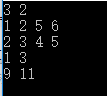4）Bellman-Ford算法（解决负权边，解决单源最短路径，前几种方法不能解决负权边）主要思想：所有的边进行n-1轮松弛，因为在一个含有n个顶点的图中，任意两点之间的最短路径最多包含n-1条边。换句话说，第1轮在对所有的边进行松弛操作后，得到从1号顶点只能经过一条边到达其余各定点的最短路径长度，第2轮在对所有的边进行松弛操作后，得到从1号顶点只能经过两条边到达其余各定点的最短路径长度，........此外，Bellman-Ford算法可以检测一个图是否含有负权回路：如果经过n-1轮松弛后任然存在dst[e[i]]>dst[s[i]]+w[i].例1：对图中含有负权的有向图，输出从1号节点到各节点的最短距离，并判断有无负权回路。#include<bits/stdc++.h>
using namespace std;
#define nmax 1001
#define inf 999999999
int n,m,s[nmax],e[nmax],w[nmax],dst[nmax];
int main ()
{
while(cin>>n>>m&&n!=0&&m!=0){
for(int i=1;i<=m;i++){
cin>>s[i]>>e[i]>>w[i];
}
for(int i=1;i<=n;i++)
dst[i]=inf;
dst=0;
for(int i=1;i<=n-1;i++){
for(int j=1;j<=m;j++){
if(dst[e[j]]>dst[s[j]]+w[j]){
dst[e[j]]=dst[s[j]]+w[j];
}
}
}
int flag=0;
for(int i=1;i<=m;i++){
if(dst[e[i]]>dst[s[i]]+w[i]){
flag=1;
}
}
if(flag) cout<<"此图有负权回路"<<endl;
else{
for(int i=1;i<=n;i++){
if(i==1) cout<<dst[i];
else cout<<' '<<dst[i];
}
cout<<endl;
}
}
}
程序运行结果如下：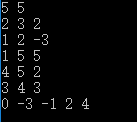5）SPFA(Shortest Path Faster Algorithm)算法是求单源最短路径的一种算法，它是Bellman-ford队列优化，它是一种十分高效的最短路算法。实现方法：建立一个队列，初始时队列里只有起始点s，在建立一个数组记录起始点s到所有点的最短路径（初始值都要赋为极大值，该点到他本身的路径赋为0）。然后执行松弛操作，用队列里的点去刷新起始点s到所有点的距离的距离，如果刷新成功且刷新的点不在队列中，则把该点加入到队列，重复执行直到队列为空。此外，SPFA算法可以判断图中是否有负权欢=环，即一个点的入队次数超过N。#include<bits/stdc++.h>
using namespace std;
int n,m,len;
struct egde{
int to,val,next;
}e;
e[len].to=to;
e[len].val=val;
len++;
}
void spfa()
{
queue<int>q;
q.push(1);
vis=1;
while(!q.empty())
{
int t=q.front();
q.pop();
vis[t]=0;
int s=e[i].to;
if(dis[s]>dis[t]+e[i].val){
dis[s]=dis[t]+e[i].val;
if(vis[s]==0){
q.push(s);
vis[s]=1;
}
}
}
}

}
int main(){
int from,to,val;
while(cin>>n>>m){
memset(vis,0,sizeof(vis));
/* for(int i=1;i<=n;i++){
dis[i]=99999999;
}*/
memset(dis,0x3f,sizeof(dis));
dis=0;len=1;
for(int i=0;i<m;i++){
cin>>from>>to>>val;
}
spfa();
for(int i=1;i<=n;i++){
cout<<dis[i]<<" ";
}
cout<<endl;
}
}

展开全文• ## 最短路径详解

万次阅读 2016-05-05 01:57:24
最短路径：一个图里有很多边，每条边有权值，两点之间的权值最小的路径。 负权回路：一个环（某点出发走了一圈还回到原点）里的权值和为负数（环里的每个权值可正可负，但和为负）。 首先，存在负权回路的图里没有...


Floyd-Warshall
原理
实现

Dijkstra
简单粗暴的原理
过程
实现

Spfa
原理
证明
实现

Bellman-Ford

最短路径：一个图里有很多边，每条边有权值，两点之间的权值最小的路径。
负权回路：一个环（某点出发走了一圈还回到原点）里的权值和为负数（环里的每个权值可正可负，但和为负）。
首先，存在负权回路的图里没有最短路，因为只要一直走这个回路就可以达到无限短。所以以下算法都是基于无负权回路的前提下。
算法验证：用HDU 2544 最短路提交能对就认为代码正确。

Floyd-Warshall

适用范围：无负权回路即可，边权可正可负，运行一次算法即可求得任意两点间最短路
时间复杂度：O(n^3)

定义dp[i][j]：i到j的最短路径，则在初始化dp的原图数据后，核心代码就这么短

void floyd() {
for (int k = 1; k <= n; ++k) {
for (int i = 1; i <= n; ++i) {
for (int j = 1; j <= n; ++j) {
dp[i][j] = min(dp[i][j], dp[i][k] + dp[k][j]);
}
}
}
}


千万别以为k代表的是除i,j外的第三个点，要这么以为的话代码中的k循环应该在最里面，  即对每对(i,j)选择一个第三点k中转，但这样结果是错的。那k代表什么？往下看。

原理

打开算法导论（英文版）第693页看看，我知道你不想看英文，所以看下面的个人理解和翻译。

假定结点集V为{1,2..n}，对于 (i, j) 这条路，我们考虑它中途经过一些结点的所有情况（这些结点都取自集合{1,2,..k}），然后定义路径p为所有情况里的最短路径（即我们要找的答案路径）。那么关于k的p的关系有两种：

k不在 最短路径p 里，即p里的点都是{1,2,..k-1}的点，则显然(i, j)经过{1,2,..k}的最短路 和 经过{1,2,..k-1}的最短路是一样的。
k在 最短路径p 里，则p里的点都是{1,2,..k}的点，那我们可以把p分为(i,k)和(k,j)，这两条分出来路径的只含{1,2,..k-1}，因为p是最短路径，而k又在p里，所以(i,k)和(k,j)都是相对于(i,j)的最短路径  【此处算导没给证明，我们先假定自己认可这个结论】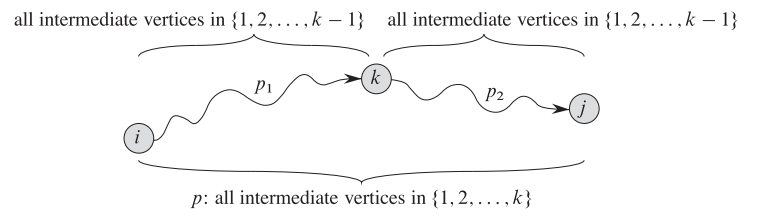根据上面的两种情况我们就可以得出递推式子

d(k)ij={wijifk=0min(d(k−1)ij,d(k−1)ik+d(k−1)kj)ifk≥1${d}_{ij}^{\left(k\right)}=\left\{\begin{array}{l}{w}_{ij}\phantom{\rule{2em}{0ex}}\phantom{\rule{2em}{0ex}}\phantom{\rule{2em}{0ex}}\phantom{\rule{2em}{0ex}}\phantom{\rule{2em}{0ex}}\phantom{\rule{2em}{0ex}}\phantom{\rule{2em}{0ex}}\phantom{\rule{1em}{0ex}}\phantom{\rule{thinmathspace}{0ex}}if\phantom{\rule{thinmathspace}{0ex}}k=0\\ min\left({d}_{ij}^{\left(k-1\right)},{d}_{ik}^{\left(k-1\right)}+{d}_{kj}^{\left(k-1\right)}\right)\phantom{\rule{2em}{0ex}}\phantom{\rule{2em}{0ex}}\phantom{\rule{1em}{0ex}}if\phantom{\rule{thinmathspace}{0ex}}k\ge \phantom{\rule{thinmathspace}{0ex}}1\end{array}${ d }_{ ij }^{ (k) }=\begin{cases} { w }_{ ij }\qquad \qquad \qquad \qquad \qquad \qquad \qquad \quad\, if\, k=0 \\ min({ d }_{ ij }^{ (k-1) },{ d }_{ ik }^{ (k-1) }+{ d }_{ kj }^{ (k-1) })\qquad \qquad \quad if\, k\ge \, 1 \end{cases}

注意k是集合大小，不是经过的点个数，k=0的时候是不经过任何中间点的情况，k>=1表示经过{1,2..k}这个集合里的点集。没看懂式子的话再看下那两种情况，看懂的话我们发现需要三维数组才能表示这种d(k)ij${d}_{ij}^{\left(k\right)}$${ d }_{ ij }^{ (k) }$ ，但在式子中我们的(k)其实只用在递推上，所以在上面代码中我们把k循环放在最外面就可以确保在计算d(k)ij${d}_{ij}^{\left(k\right)}$${ d }_{ ij }^{ (k) }$ 前 dp[i][j]存的是d(k−1)ij${d}_{ij}^{\left(k-1\right)}$${ d }_{ ij }^{ (k-1) }$，同理 d(k−1)ik${d}_{ik}^{\left(k-1\right)}$${ d }_{ ik }^{ (k-1) }$ 和d(k−1)ik${d}_{ik}^{\left(k-1\right)}$${ d }_{ ik }^{ (k-1) }$ 也是一样。这点也就类似背包的二维压成一维。

实现

dp数组对于不存在的边初始化为无穷大，但直接用INT_MAX的话在dp[i][k] + dp[k][j]的时候溢出，所以精确来说设置 为比全部路径的最大值大一点就行，如10条最大1000的边则设置为10*1000+1，但为写代码方便就用0x3f3f3f3f (大概10亿）比较适合。
dp[i][i]初始化为0，即使就做题而言可能不会WA。

#include <string.h>
#include <iostream>
using namespace std;
const int maxn = 105;
const int inf = 0x3f3f3f3f;
int n,m;
int dp[maxn][maxn];

void floyd() {
for (int k = 1; k <= n; ++k) {
for (int i = 1; i <= n; ++i) {
for (int j = 1; j <= n; ++j) {
dp[i][j] = min(dp[i][j], dp[i][k] + dp[k][j]);
}
}
}
}

int main(){
int a,b,c;
while(cin>>n>>m,n||m){
memset(dp,inf,sizeof(dp));
for (int i = 1; i <= n; ++i){
dp[i][i] = 0;       //不是必要
}
for (int i = 1; i <= m; ++i){
cin>>a>>b>>c;
dp[a][b] = dp[b][a] = c;
}
floyd();
cout<<dp[n]<<endl;
}
return 0;
}

Dijkstra

通俗翻译为迪杰斯特拉算法
- 适用范围：无负权回路，边权必须非负，单源最短路
- 时间复杂度：优化前O(n2${n}^{2}$$n ^ 2$)

简单粗暴的原理

更新：2018-02-21
求t 点到s点的距离，假设距离s点最近的点p1距离为L，那么这个点一定是最短的，因为不可能有比直达最近的点还近的路，那么选它没错。

然后把s和点p1看成一个点S’，再同理选距离S’最近的点（其实这里实际求的是距离最开始的源点s），就这样一直重复操作贪心下去即可。

其中在选了p1之后我们要更新所有p1点相邻点到s点的最短距离，因为选p1点那么可能经过p1点到s点比原本的点直接到s点更近。

注意求点距离的时候求的是距离源点s最近，不是距离集合S’最近，距离集合S’最近就是最小生成树Prim算法了。

过程

数组dis[u]表示u到s点的最短距离。
我们一直找点u = min{ dis[k] , k点未访问 }，这个点就是最短路上的点，然后根据其他点v跟u点的关系去更新下dis[v]，不断重复找和更新即可。
dis[s]=0将源点加入最短路，然后循环n-1次每次找出一个最短路上的点，找的方法是直接找出剩下的点中dis[ ]最小的那个点u，u点就是最短路上的点，然后看看其他点v到s点的距离会不会因为这个u点的加入而改变，即若dis[v] > dis[u] + distance[u][v] 则更新dis[v]为 dis[u] + distance[u][v]。

实现

最基础的实现是邻接矩阵（二维数组），然后在找最小的dis[]部分可以用优先队列/最小堆优化查找速度。

#include <cstring>
#include <iostream>
using namespace std;

const int maxn = 105;
const int inf = 0x3f3f3f3f;
int dis[maxn];
bool vis[maxn];
int map_dis[maxn][maxn];
int n,m;
int dijkstra(int s, int t) {
memset(vis, false, sizeof(vis));
for (int i = 1; i <= n; ++i) {      //初始化各点到s点的距离
dis[i] = map_dis[s][i];
}
dis[s] = 0, vis[s] = true;

for (int i = 0; i < n - 1; ++i) {   //除s点外找n-1个点
int u, tmin = inf;
for (int j = 1; j <= n; ++j){   //找min{dis[]}
if(!vis[j] && dis[j] < tmin){
tmin = dis[j];
u = j;
}
}
// if(tmin == inf) return -1;   //无最短路
vis[u] = true;                  //进入T集合
for (int v = 1; v <= n; ++v){   //更新相邻点
if(!vis[v] && dis[u] + map_dis[u][v] < dis[v]){
dis[v] = dis[u] + map_dis[u][v];
}
}
}
return dis[t];
}

int main() {
int a, b, c;
while (cin >> n >> m, n || m) {
memset(map_dis,inf,sizeof(map_dis));
for (int i = 1; i <= m; ++i) {
cin >> a >> b >> c;
map_dis[a][b] = map_dis[b][a] = c;
}
cout << dijkstra(1,n) << endl;
}
return 0;
}

Spfa

Shortest Path Faster Algorithm，是国内原创算法，作者：西南交通大学段凡丁。
- 适用范围：边权可正可负，单源最短路，还可以判断图中有无负权回路
- 时间复杂度：O(kE)，k非常数，一般认为是所有点的平均入列次数且k一般小于等于2

原理

算法思路很简单，将源点加入队列，然后不断从队列中弹出顶点u，遍历u的邻接点v进行松弛更新（若dis[v] < dis[u] + distance[u][v] 则更新dis[v]为dis[u] + distance[u]），更新后如果v点不在队列里则进入队列。

证明

每次将点放入队尾，都是经过松弛操作达到的。换言之，每次的优化将会有某个点v的最短路径估计值dis[v]变小。所以算法的执行会使dis越来越小。由于我们假定图中不存在负权回路，所以每个结点都有最短路径值。因此，算法不会无限执行下去，随着d值的逐渐变小，直到到达最短路径值时，算法结束，这时的最短路径估计值就是对应结点的最短路径值。（证毕）

实现

算法思路本身是队列，不过也可以用栈。
队列方案判断负权环：如果某点进入队列的次数 > n次。
栈方案判断负权环：如果某点进入栈的次数 >= 2，栈方法判负环比较高效。

#include <cstdio>
#include <cstring>
#include <string>
#include <stack>
#include <queue>
#include <iostream>
#include <algorithm>
using namespace std;

const int maxn = 105;
const int maxm = 10000;
const int inf = 0x3f3f3f3f;
struct Edge{
int v, w, next;
} edge[maxm * 2];
int cnt;
void add_edge(int u, int v, int w){ //邻接表前插法
edge[cnt].v = v; edge[cnt].w = w; edge[cnt].next = head[u]; head[u] = cnt++;
}
void init(int n){
cnt = 0;
memset(inq, 0, sizeof(inq));
memset(dis, inf, sizeof(dis));
}
int spfa(int s, int t){
queue<int>q;
q.push(s);
dis[s] = 0;
inq[s] = 1;
while (!q.empty()){
int u = q.front(); q.pop();
inq[u] = 0;
for (int i = head[u]; i != -1; i = edge[i].next) {
int v = edge[i].v;
int w = edge[i].w;
if (dis[v] > dis[u] + w){
dis[v] = dis[u] + w;
if (!inq[v]){
inq[v] = 1;
q.push(v);
}
}
}
}
return dis[t];
}

int main() {
int n, m, a, b, c;
while (cin >> n >> m, n || m) {
init(n);
for (int i = 0; i < m; ++i) {
cin >> a >> b >> c;
}
cout << spfa(1, n) << endl;
}
return 0;
}

队列式判断负权环

bool spfa(int s, int t){
queue<int>q;
q.push(s);
dis[s] = 0;
inq[s] = 1;
times[s]++;
while (!q.empty()){
int u = q.front(); q.pop();
inq[u] = 0;
for (int i = head[u]; i != -1; i = edge[i].next) {
int v = edge[i].v;
int w = edge[i].w;
if (dis[v] > dis[u] + w){
dis[v] = dis[u] + w;
if (!inq[v]){
inq[v] = 1;
q.push(v);
times[v]++;
if(times[v] > n){
return false;
}
}
}
}
}
return true;
}

Bellman-Ford

适用范围：边权可正可负，单源最短路，还可以判断图中有无负权回路
时间复杂度：O(VE)，巨慢

Dijkstra算法以贪心法选取未被处理的具有最小权值的节点，然后对其的出边进行松弛操作；而Bellman-Ford简单地对所有边进行松弛操作

BELLMAN-FORD(G, w, s)
1   INITIALIZE-SINGLE-SOURCE(G, s)
2   for i ← 1 to |V[G]| - 1
3       do for each edge (u, v) ∈ E[G]
4              do RELAX(u, v, w)
5   for each edge (u, v) ∈ E[G]
6       do if d[v] d[u] + w(u, v)
7             then return FALSE
8  return TRUE

因为效率实在是很低，就不多介绍了
展开全文算法 dijkstra Floyd
• 迪杰斯特拉(Dijkstra)算法是典型最短路径算法，用于计算一个节点到其他节点的最短路径。 它的主要特点是以起始点为中心向外层层扩展(广度优先搜索思想)，直到扩展到终点为止。算法思想 每次找到离源点最近的一个...dijkstra
• 最短路径 最小生成树是以无向带权图为基准，而最短路径则是以加权有向图为基准 最短路径树 给定一幅加权有向图和一个顶点s。 以s为起点的一棵最短路径树是图的一幅子图，它包含s和从s可达的所有顶点。 根节点为s，...算法
• ## 最短路径

千次阅读 2019-09-10 16:16:07
在带权图中，把一个顶点从v0到图中任意一个顶点vi的一条路径（可能不止一条）所经过边上的权值之和，定义为该路径的带权路径长度，把带权路几个长度最短的那条路径称为最短路径。 带权有向图的最短路径分为两类 ： ...迪杰特斯拉 Floyd
• 最短路径问题 题目 平面上有n个点(N&amp;amp;amp;lt;=100)，每个点的坐标均在-10000~10000之间。其中的一些点之间有连线。若有连线，则表示可从一个点到达另一个点，即两点间有通路，通路的距离为两点直线的...
• 最短路径 在非网图中，最短路径是指两个顶点之间经历的边数最少的路径，路径上的第一个顶点称为源点，最后一个顶点称为终点。 在网图中，最短路径是指两点之间经历的边上的权值之和最少的路径。 Dijkstra算法 ...算法 数据结构
• ## 漫画：图的 “最短路径” 问题

千次阅读 多人点赞 2019-04-08 08:48:00
————— 第二天 —————如何遍历呢？第一层，遍历顶点A：第二层，遍历A的邻接顶点B和C：第三层，遍历顶点B的邻接顶点D、E，遍历顶点C的邻接顶点F：第四层，遍历...
• ## 最短路径四大算法

万次阅读 多人点赞 2017-08-19 10:14:22
熟悉的最短路算法就几种：bellman-ford，dijkstra，spfa，floyd。首先说明一点，就是关于负环的问题。 bellman-ford可以用于边权为负的图中，图里有负环也可以，如果有负环，算法会检测出负环。...
• 本文总结了图的几种最短路径算法的实现：深度或广度优先搜索算法，弗洛伊德算法，迪杰斯特拉算法，Bellman-Ford算法 1），深度或广度优先搜索算法（解决单源最短路径） 从起始结点开始访问所有的深度遍历路径...迪杰斯特拉 算法
• 一、Dijkstra算法 1、单源点的最短路径问题：给定带权有向图G和源点v，求从v到G中其余各顶点的最短路径。 我们用一个例子来具体说明迪杰斯特拉算法的流程。 定义源点为 0，dist[i]为源点 0 到顶点 i 的最短...数据结构 Floyd算法
• Dijkstra算法研究的是从初始点到其他每一结点的最短路径 而Floyd算法研究的是任意两结点之间的最短路径 以下图为例，首先介绍Dijstra的原理 红字为各结点的编号，蓝字为各结点之间的距离 首先定义几个变量 ...MATLAB
• 本设计以VC++6.0作为程序开发环境，C语言作为程序开发语言，详细介绍了最短路径的求解算法及其C语言实现过程。系统主要实现了图的创建、单源点最短路径的计算功能。依照本系统可以解决实际生活中许多路径选择问题，...
• 问题：根据每条边的权值，求出从起点s到其他每个顶点的最短路径最短路径的长度。 说明：不考虑权值为负的情况，否则会出现负值圈问题。 s：起点 v：算法当前分析处理的顶点 w：与v邻接的顶点 dvdvd_v：从s到v...
• 最短路径的概念 最短路径的问题是比较典型的应用问题。在图中，确定了起始点和终点之后，一般情况下都可以有很多条路径来连接两者。而边或弧的权值最小的那一条路径就称为两点之间的...Dijkstra算法介绍 Dijkst...数据结构 Java
• ## 单源最短路径---Dijkstra算法

万次阅读 多人点赞 2018-01-13 21:38:29
他想要到3去，请问最短路径是多少。 很容易得到该图的邻接矩阵。我们建立一个二维数组a。a[i][j]，i表示 起点，为行，j表示终点，为列。将相应的权值传入其中，如果从一个 点到另一个点不通，就认为其...算法 详解 c++
• 这个算法用于解决图中单源最短路径问题。所谓单源节点是指给定源节点，求图中其它节点到此源节点的最短路径。如下图所示：给定源节点a，求节点b到a的最短距离。 (图来自于参考资料2) 那么如何寻找？还是...
• 前言 今天将给大家介绍的是图论算法中的另外一个基础部分——最短路径算法；其中又分为无权最短路径，单源最短路径，具有负边的最短路径以及无圈图等；而这次将介绍常见的两个——无权最短路径以及单源最短路径。接...C 数据结构
• 不知道为什么，花了好长时间弄懂了，也写了一遍，又遇到时还是出错了，今天再次写它，心里没那么怕了，耐心研究，懂了之后会好开心的，哈哈Dijkstra算法：图G 如图：若要求从顶点1到其余各顶点的最短路径，该咋求；...
• 一个求单源最短路径的问题。给定有向带权图 G =(V, E ), 其中每条边的权是非负实数。此外,给定 V 中的一个顶点, 称为源点。现在要计算从源到所有其他各顶点的最短路径长 度,这里路径长度指路上各边的权之和。 2...贪心算法
• Dijkstra(迪杰斯特拉)算法是典型的单源最短路径算法，用于计算一个节点到其他所有节点的最短路径。主要特点是以起始点为中心向外层层扩展，直到扩展到终点为止。本实例实现了求最小路径的权值还能绘出最小路径的走法...
• 最近在做算法题的时候总是遇到Dijkstra相关的题目，...关于Dijkstra的理论知识可以参考这篇博客：最短路径问题-Dijkstra算法详解 Dijkstra算法 Dijkstra算法往往和dfs结合在一起考，因此这里给出一个求解基础Dijk...
• 本文介绍时间复杂度为O(v^2)的Dijkstra算法，但只适用于没有负权重边的有向图。 基本上所有计算图的最短路径的算法都基于一个性质：一条最短路径的子路径肯定也是一条最短路径。该性质用反证法就可以轻易证明。反...
• 设S为已找到从v出发的最短路径集合，它的初始状态为空集，设T=V-S为图中剩余顶点集合，编程时可以使用长度为n（n为图的顶点数目）数组visited表示集合S，visited[i]=1表示第i个顶点属于集合S，visited...
• 因为之前刚写过最小生成树算法，刚开始看到Dijkstra算法的时候，因为要求各点到源点的最短距离，会想着直接从最小生成树的也是每找到最短的距离点加进来，但是后面仔细想了下，又翻了下定义，得到 最短路径是一个图...c#
• Dijkstra算法 1.定义概览 Dijkstra(迪杰斯特拉)算法是典型的单源最短路径算法，用于计算一个节点到其他所有节点的最短路径。主要特点是以起始点为中心向外层层扩展，直到扩展到终点为止。数据结构
• 如果所采用的实现方式合适，Dijkstra算法的运行时间要低于前面所说的Bellman_Ford算法，但是Dijkstra算法要求图中没有负边。Dijkstra算法在运行过程中维持的关键信息是一组结点集合S。从源结点s到该集合中每个结点...Java 算法
• Dijkstra算法——引入 （1）定义:从图中的某个顶点出发到达另外一个顶点的所经过的边的权重和最小的一条路径，称为最短路径； （2）算法应用：移动机器人在路径规划中，得知起始点和终止点，在众多的可行驶路径中......